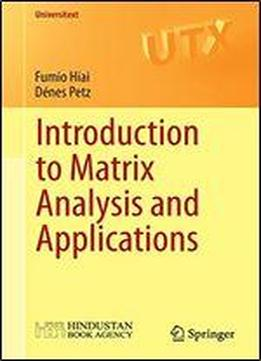Introduction To Matrix Analysis And Applications by Fumio Hiai / 2014 / English / PDF

Matrices can be studied in different ways. They are a linear algebraic structure and have a topological/analytical aspect (for example, the normed space of matrices) and they also carry an order structure that is induced by positive semidefinite matrices. The interplay of these closely related structures is an essential feature of matrix analysis. This book explains these aspects of matrix analysis from a functional analysis point of view. After an introduction to matrices and functional analysis, it covers more advanced topics such as matrix monotone functions, matrix means, majorization and entropies. Several applications to quantum information are also included. Introduction to Matrix Analysis and Applications is appropriate for an advanced graduate course on matrix analysis, particularly aimed at studying quantum information. It can also be used as a reference for researchers in quantum information, statistics, engineering and economics.

views: 237